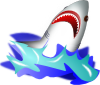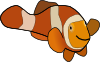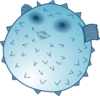Click here for the Problem Extension Worksheet version of the Problems of the Week.
Click here for an MS Word version of the Problems of the Week.
Click here for the Canadian Problem Extension Worksheet version of the Problems of the Week.
Click here for a Canadian MS Word version of the Problems of the Week.Lower Elementary:
Question: A shark eats 10 fish on Monday, 9 fish on Tuesday, 8 fish on Wednesday, and so on. If the pattern continues, how many fish does the shark eat over the course of 1 whole week?
Answer: 49 fish
Solution: The pattern is that the shark eats one fewer fish each day. So, since there are 7 days in a week, the shark eats 10 + 9 + 8 + 7 + 6 + 5 + 4 = 49 fish.Upper Elementary:
Question: A giant pacific octopus weighs 110 pounds. A blue-ringed octopus weighs 4 ounces. How many blue-ringed octopuses weigh the same amount as a giant pacific octopus?
Answer: 440 blue-ringed octopuses
Solution: A pound is 16 ounces, so it takes 16 ÷ 4 = 4 blue-ringed octopuses to make up a pound. So, 4 × 110 = 440 blue-ringed octopuses weigh the same as a 110-pound giant pacific octopus.Middle School:
Question: An anemone catches 10 pounds of food over the course of a week and consumes 62.5% of it. A school of clownfish that lives with the anemones eat 3/8 of the anemone’s uneaten prey. What percentage of the anemone’s food goes uneaten?
Answer: 23.4375%
Solution: Since 62.5% of the anemone’s prey is eaten, that means 100% – 62.5% = 37.5% of it is not eaten. The clownfish eat 3/8 of the leftovers, so they leave 1 – 3/8 = 5/8 uneaten. So, since 5/8 = 62.5%, we need to find 62.5% of 37.5%. We do that by multiplying 0.625 × 0.375 = 0.234375 = 23.4375%Algebra and Up:
Question: Some species of pufferfish draw circles in the sandy seafloor to communicate. If a pufferfish draws a circle whose diameter is 12 times the length of the fish’s body, then how many times the length of the fish is the circumference of the circle?
Answer: 12π
Solution: The circumference of a circle is its diameter multiplied by π. If we say that P is the length of the pufferfish, then we can then say that the diameter is 12P, so the circumference is 12Pπ. If we divide out the length of the pufferfish, we find that the circumference is 12π times the length of the pufferfish.